×#### Thank you for registering.

One of our academic counsellors will contact you within 1 working day.

Click to Chat

1800-1023-196

+91-120-4616500

CART 0

• 0

MY CART (5)

Use Coupon: CART20 and get 20% off on all online Study Material

ITEM
DETAILS
MRP
DISCOUNT
FINAL PRICE
Total Price: Rs.

There are no items in this cart.
Continue Shopping• Complete Physics Course - Class 11
• OFFERED PRICE: Rs. 2,968
• View Details

```Continuous Charge Distribution

Introduction to Continuous Charge Distribution

What is Continuous Charge Distribution?

Linear Charge Density

Surface Charge Density

Volume Charge Density

Introduction to Continuous Charge Distribution

With the help of Coulomb’s Law and Superposition Principle, we can easily find out the electric field due to the system of charges or discrete system of charges. The word discrete means every charge is different and has the existence of its own. Suppose, a system of charges having charges as q1, q2, q3……. up to qn. We can easily find out the net charge by adding charges algebraically and net electric field by using the principle of superposition. This is because:

Discrete system of charges is easier to solve

Discrete system of charges do not involve calculus in calculations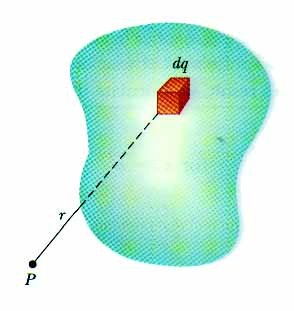Image 1: A system in which charge is distributed over a conductor, is called continuous charge distribution system

But how to calculate electrostatics terms in continuous charge system? For an Example if there is a rod with charge q, uniformly distributed over it and we wish to find the electric field at some distance ‘r’ due it. It would be illogical and irrelevant to simply add electric field using principle of superposition as the charge is uniformly distributed over the rod. So we take a small element of the rod and integrate it with proper limits.

We consider element, based on how density of charge is centered on the material or object. If the charge is uniformly distributed over the surface of the conductor, then it is called Surface Density. If the charge varies linearly along the length of the conductor, then it is called Linear Charge Density. And if the charge changes with volume of the conductor, then it is called Volume Charge Density.

What is Continuous Charge Distribution?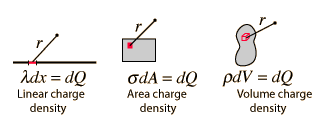Image 2: Types of Charge Distribution

The continuous charge distribution system is a system in which the charge is uniformly distributed over the conductor. In continuous charge system, infinite numbers of charges are closely packed and have minor space between them. Unlikely from the discrete charge system, the continuous charge distribution is uninterrupted and continuous in the conductor. There are three types of the continuous charge distribution system.

Linear Charge Distribution

Surface Charge Distribution

Volume Charge Distribution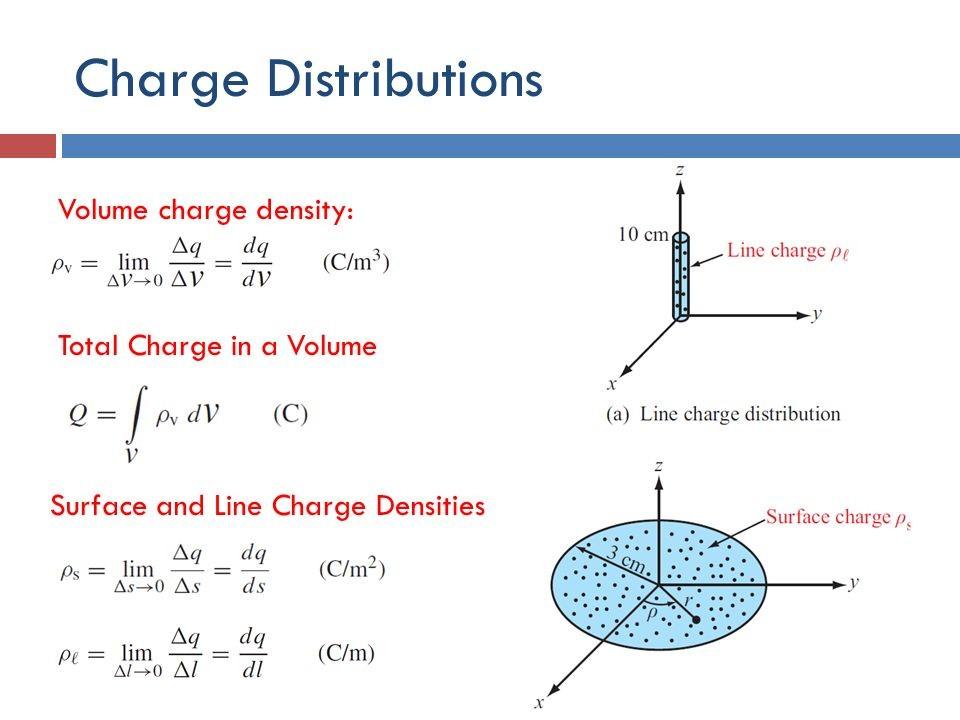Image 3: Types of charge distribution system

Linear Charge Density

When the charge is non-uniformly distributed over the length of a conductor, it is called linear charge distribution. It is also called linear charge density and is denoted by the symbol λ (Lambda).

Mathematically linear charge density is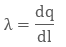The unit of linear charge density is C/m. If we consider a conductor of length ‘L’ with surface charge density λ and take an element dl on it, then small charge on it will beSo, the electric field on small charge element dq will be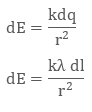To calculate the net electric field we will integrate both sides with proper limit, that is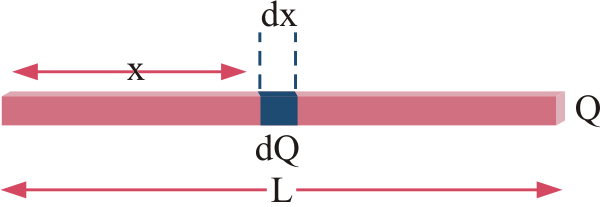Image 4: We take small element x and integrate it in case of linear charge density

Surface Charge Density

When the charge is uniformly distributed over the surface of the conductor, it is called Surface Charge Density or Surface Charge Distribution. It is denoted by the symbol σ (sigma) symbol and is the unit is C / m2.

It is also defined as charge/ per unit area. Mathematically surface charge density is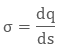where dq is the small charge element over the small surface ds. So, the small charge on the conductor will beThe electric field due to small charge at some distance ‘r’ can be evaluated asIntegrating both sides with proper limits we get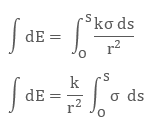Volume Charge Density

When the charge is distributed over a volume of the conductor, it is called Volume Charge Distribution. It is denoted by symbol ρ (rho). In other words charge per unit volume is called Volume Charge Density and its unit is C/m3. Mathematically, volume charge density is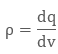where dq is small charge element located in small volume dv. To find total charge we will integrate dq with proper limits. The electric field due to dq will beIntegrating both sides with proper limits we get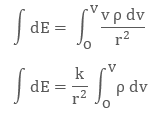Image 5: We can easily find electric field in different geometries using charge distribution system

Watch this Video for more reference

Continuous Charge Distribution
```### Course Features

• 101 Video Lectures
• Revision Notes
• Previous Year Papers
• Mind Map
• Study Planner
• NCERT Solutions
• Discussion Forum
• Test paper with Video Solution